Matrix Inversions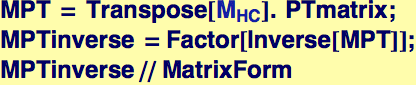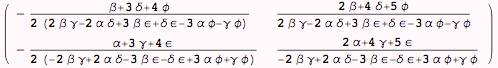The denominators are related to the determinant---if the determinant vanishes, then the inverse matrix is not defined.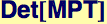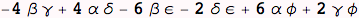Checking to see if the the inverse multiplied by the original matrix is the identity matrix: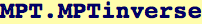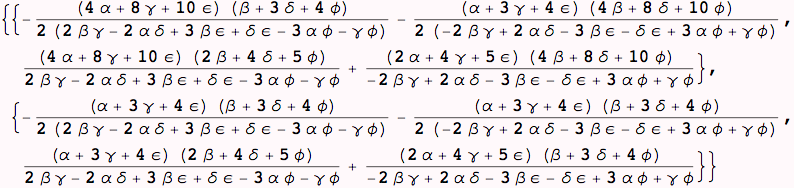It is not obvious unless simplified...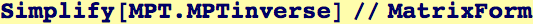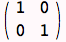Created by Wolfram Mathematica 6.0  (31 August 2007)